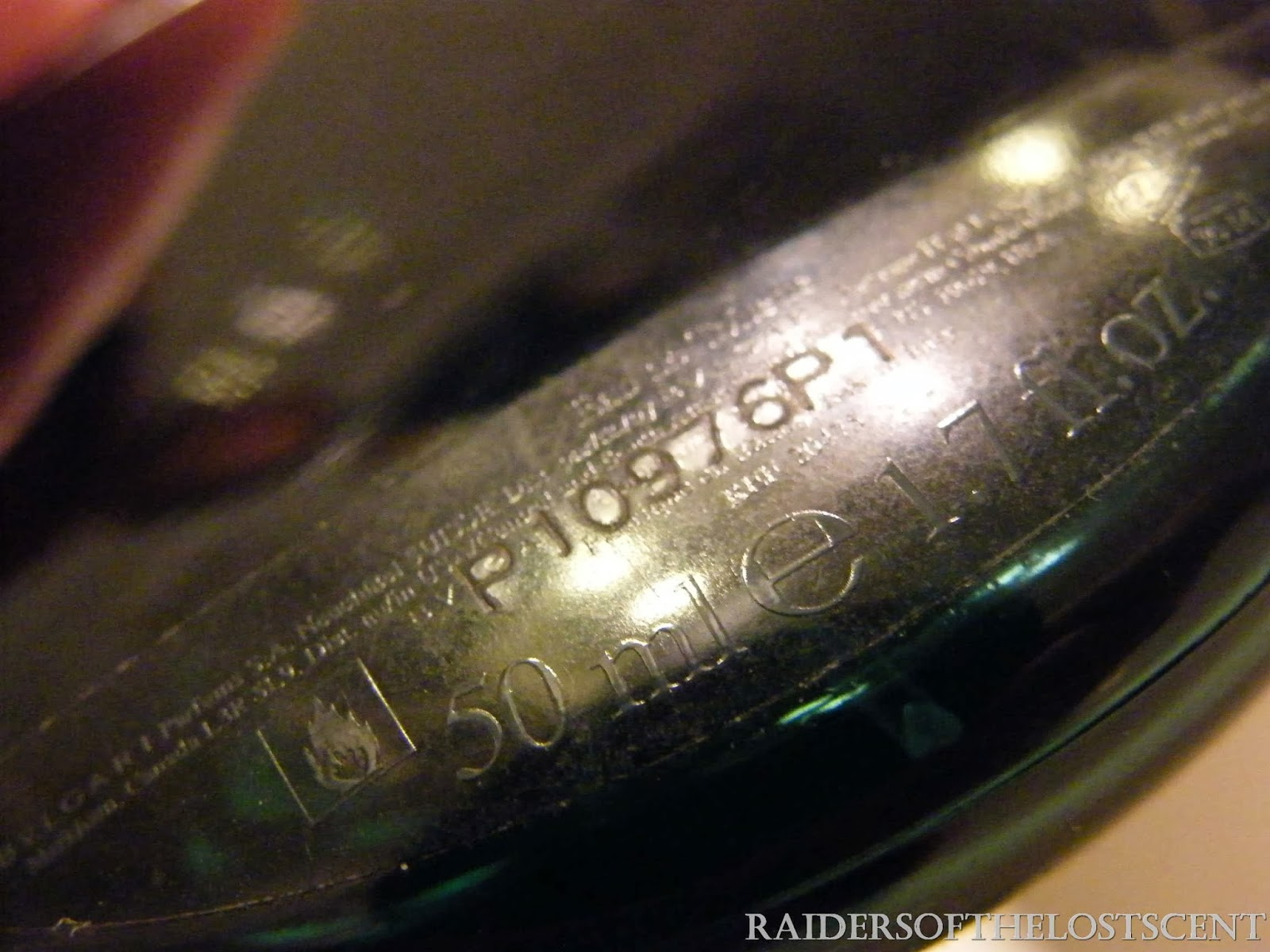## martedì 12 novembre 2013

### How to recognize BVLGARI Perfumes

HOW TO RECOGNIZE BVLGARI PERFUMES.

Previously we recognized, batch-by-batch, year-by-year:
-GUERLAIN perfumes ( here )
-YVES SAINT LAURENT perfumes ( here )
-CHRISTIAN  DIOR perfumes  ( here )
-ARMANI Perfumes ( here
-CHANEL ( here )
Now we will consider another House: Bulgari

Bulgari Perfumes are difficult in putting a date, and it is impossible to get a sort of quick "batch-number table", so you have to consider each different situation, case-by-case.
Why it's so difficult? Because there is a complicate way of coding.

Bulgari batch numbers use usually 7-or-8 digits, and apparently the code is unexplicable.
For example, here is a Bulgari batch number:

10C63A1W

Don't worry! A solution exists!

First thing to do: check the THIRD digit from the left: is it a LETTER or a NUMBER?
1- if it's a letter, it means = since 2010
2- if it's a number, it means = before 2010.

first case:
batch number is 10C63A1W
is the third digit (starting from the left) a letter? YES
so: A =2010  B=2011   C=2012   D=2013
You must check the two numbers on left and right of that letter.
In this case : "0C6", the two numbers are : "06".
This means = June 2012

second case:
Batch number is 21A15AT1
third digit is -again- a letter: this is "A".
so: A =2010  B=2011  C=2012   D=2013
This means year 2010.
two numbers on left and right are : "1A1" , so  "11"
this means = November, 2010.

Things are very complicate now......
In this case, third digit is NOT a letter, but a number.

Pay attention:  reading the batch nuymber, you MUST arrive to the last "letter and number", and REMOVE this "couple", plus all following digits.
example:  batch code is A31517B3
(third digits is not a letter, ok, so it's before 2010....)
Now look carefully: the last "letter and number" is "7B". Now remove these two, AND all digits (i.e: remove 7B3).
now the batch will be: A3151
consider last three numbers : the middle is year, the edges are the month: it's "151"  = "5" and "11" = November, 2005.

Another case:
batch number P10359PW2
(third digit is not a letter, so it's "before 2010"....)
check last "letter and number" (it is "9P"), and remove this couple and all following digits ( i.e: remove "9PW2" )
now the batch is: P1035
consider last three numbers: "035" , the middle it's year, the edges are the month:
"3" and "05" = May, 2003

The most of Bulgari Perfumes was produced after year 1999, so, since numbers can repeat every decade, you should get an help knowing the year of launch, clues on the boxes, etc..

At beginning it's difficult, with some practice it will be easy!

TUTORIAL PICTURESBulgari Aqua for Man, Batch: P10976P1Remove last  "letter and number" and following digits, i.e. "6P1"; new batch is P1097 nowConsider only last three numbers: it's "097"Middle is YEAR, edges are MONTH, so = September, 2009Bulgari pour Homme, Batch code is 10452C1Remove last "letter and number" ( "2C" and following digits ),new batch is "1045"Consider only last three letters: "045"Middle is YEAR, edges are MONTH:so, it's May, 2004Bulgari Man, batch number: 01A26E2Easy case: is the third digit a letter? Yes! it's "A", so it's 2010.Numbers on left and right are 1 and 2, so, it's : December, 2010Bulgari Blv, Batch number is 00248AF1remove last "letter and number", i.e.  "8A" and subsequent digits.Now the batch code is: 0024consider only last three numbers, it's "024".Middle is YEAR, edges are MONTH, so: April, 2002Batch number is 20191AF1Remove "1AF1", new batch code is 2019consider only last three numbers: "019"Middle is YEAR, edges are MONTH, so: September 2001Bulgari Black, Batch number is : 00186V1remove 6V1, new batch is "0018"consider last three numbers: "018"Middle is YEAR, edges are MONTH, so= August 2001.

#### 2 commenti:

1.How to decipher batch code "WB 61931 E" ?

1.Hi,
if you send a picture it could be easier to verify.